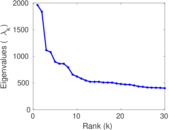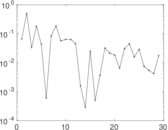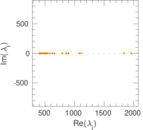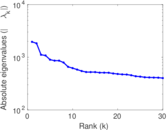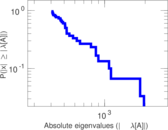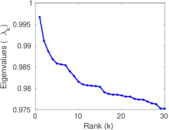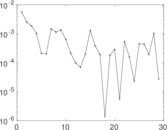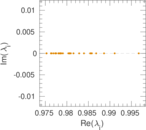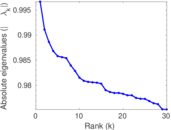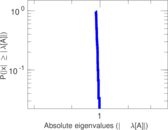This network consists of the wikilinks of the Wikipedia in the Arabic language (ar). Nodes are Wikipedia articles, and directed edges are wikilinks, i.e., hyperlinks within one wiki. In the wiki source, these are indicated with [[double brackets]]. Only pages in the article namespace are included.

 Code `War` Internal name `wikipedia_link_ar` Name Wikipedia links (ar) Data source http://dumps.wikimedia.org/ AvailabilityDataset is available for download Consistency checkDataset passed all tests Category Hyperlink network Node meaning Article Edge meaning Wikilink Network format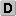Unipartite, directed Edge typeUnweighted, no multiple edges ReciprocalContains reciprocal edges Directed cyclesContains directed cycles Loops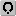Contains loops

## Statistics

 Size n = 1,073,810 Volume m = 66,458,548 Loop count l = 1,857 Wedge count s = 110,382,991,464 Claw count z = 2,394,063,851,468,089 Cross count x = 7.727 64 × 1019 Triangle count t = 6,957,262,594 Maximum degree dmax = 186,314 Maximum outdegree d+max = 2,811 Maximum indegree d−max = 186,293 Average degree d = 123.781 Size of LCC N = 1,073,380 Size of LSCC Ns = 685,690 Relative size of LSCC Nrs = 0.638 558 Diameter δ = 12 50-Percentile effective diameter δ0.5 = 3.033 30 90-Percentile effective diameter δ0.9 = 3.933 05 Median distance δM = 4 Mean distance δm = 3.519 12 Balanced inequality ratio P = 0.163 863 Outdegree balanced inequality ratio P+ = 0.163 444 Indegree balanced inequality ratio P− = 0.172 415 Degree assortativity ρ = −0.028 655 1 Degree assortativity p-value pρ = 0.000 00 Clustering coefficient c = 0.189 085 Directed clustering coefficient c± = 0.893 081 Operator 2-norm ν = 1,988.64 Cyclic eigenvalue π = 1,960.02 Reciprocity y = 0.626 567 Non-bipartivity bA = 0.802 915 Normalized non-bipartivity bN = 0.006 391 85 Algebraic non-bipartivity χ = 0.086 717 9 Spectral bipartite frustration bK = 0.000 253 465

## Plots

### Degree distribution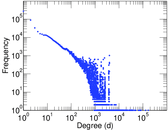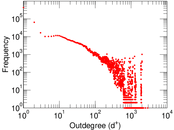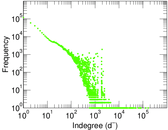### Cumulative degree distribution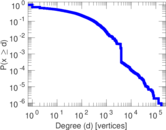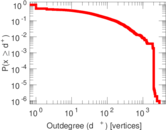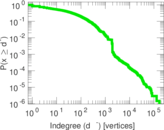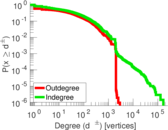### Hop distribution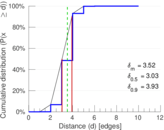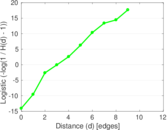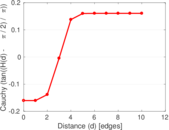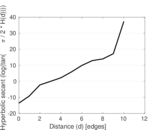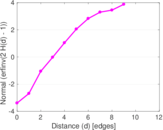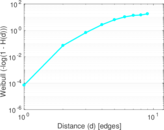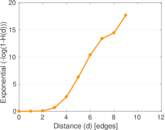### In/outdegree scatter plot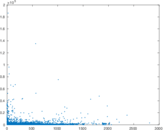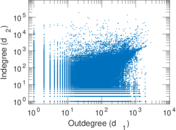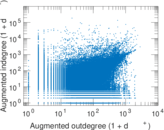### Matrix decompositions plots